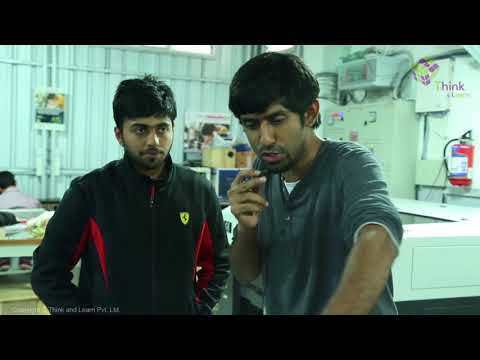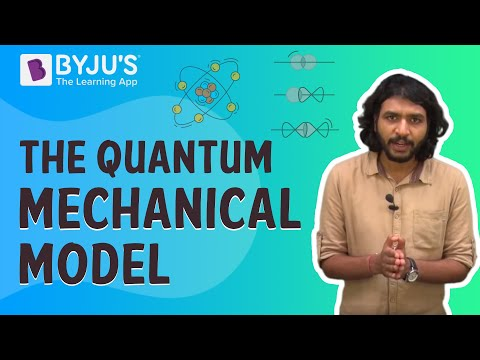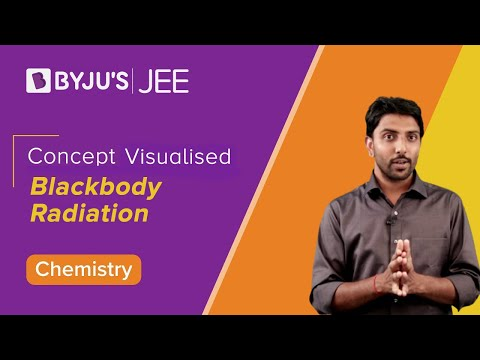Jet Set Go! All about Aeroplanes Jet Set Go! All about Aeroplanes

# Quantum Mechanics Questions

Quantum mechanics’ history is an important part of the history of modern physics. A group of physicists at the University of Göttingen, including Max Born, Wolfgang Pauli, and Werner Heisenberg, coined the term “Quantum Mechanics” in the early 1920s. At the most fundamental level, matter and radiation are both made up of waves and particles. Scientists gradually recognised that matter has wave-like properties and radiation has particle-like properties provided the impetus for the development of quantum mechanics.

 Definition: Quantum mechanics is a branch concerned with the behaviour of matter and light at the subatomic and atomic levels. It makes an attempt to explain the properties of atoms and molecules, as well as their fundamental particles such as protons, neutrons, electrons, gluons, and quarks. Particle properties include their interactions with one another as well as their interactions with electromagnetic radiation.

## Quantum Mechanics Chemistry Questions with Solutions

Q1. Choose the wrong statement about the spin of an electron, according to quantum mechanics:

a) It is related to intrinsic angular momentum.

b) Spin is the rotation of an electron about its own axis.

c) Value of the spin quantum number of electrons must not be 1.

d) +1/2 value of spin quantum number represents up spin.

Correct Answer. (b) Spin is the rotation of an electron about its own axis.

Explanation. According to quantum mechanics, an electron’s spin is related to intrinsic angular momentum; therefore, the value of a spin quantum number cannot be 1 because its -½ and +½ value represents the spin of an electron.

Q2. The Quantum Mechanical Model of the atom was proposed by:

a) Louis de Broglie

b) Erwin Schrodinger

c) Neil Bohr

d) Werner Heisenberg

Q3. The wavelength of the matter waves is independent of:

a) mass

b) velocity

c) charge

d) momentum

Q4. Assuming the velocity to be the same, which particle is having longest wavelength

a) an electron

b) a proton

c) a neutron

d) an α-particle

Q5. The uncertainty principle states that the error in measurement is due to-

a) dual nature of particles

b) due to the small size of particles

c) due to large size of particles

d) due to error in measuring instrument

Correct Answer: (b) dual nature of particles.

Q6. If the uncertainty in the velocity of a moving object is 1.0×10–6 ms–1 and the uncertainty in its positions is 58 m, the mass of this object is approximately equal to that of:

a) deuterium

b) lithium

c) electron

d) helium

Q7. Define the law of Stefan-Boltzmann.

This law states that the radiant energy (E) of the body is directly proportional to the fourth power of the temperature (T) of the body.

E ∝ T4

W = σT4

Where σ = Stefan Constant, given by

$$\begin{array}{l}\sigma =\frac{2\Pi ^{5}K_{B}^{4}}{15h^{3}c^{2}}\end{array}$$

Q8. Mention the uses of an electron microscope.

Answer. The uses of an electron microscope are as follows-

• It has numerous applications in biology, metallurgy, physics, chemistry, medicine, and engineering.
• It is used to determine the complicated crystal structures.
• It is employed in the investigation of celluloid.
• It is used to investigate the structure of microorganisms such as viruses and bacteria.

Q9. Explain Planck’s hypothesis or what are the postulates of Planck’s quantum theory?

• Electrons in a black body are assumed to be simple harmonic oscillators.
• The oscillators will not continuously emit energy.
• They emit radiation in discrete quanta of magnitude ‘hɣ’.
• E = nhɣ, where n=1, 2, 3,…..

Q10, What is the wavelength associated with a photon of a light with the energy is 3.6 ×10–19 J?

Answer. E = h𝝁 = hc/λ

$$\begin{array}{l}3.6\times 10^{-19}J=\frac{\left ( 6.626\times 10^{-34}Js \right )\left ( 3\times 10^{8}ms^{-1} \right )}{\lambda }\end{array}$$

λ = 0.55 × 10–7 m = 550 nm.

Q11. Describe Planck’s three experimental observations that explain the photoelectric effect.

Answer. “When a specific frequency of light shines on a clean metal surface, electrons are ejected from the metal.” Experiment results show that:

1. The number of electrons ejected is proportional to the light intensity.
2. The frequency of the ejected light is proportional to the kinetic energy of the ejected electrons.
3. If the frequency of the light is less than a certain value, known as the threshold frequency, no electrons can be ejected ( nuO ).

Q12. What is the difference between classical and quantum mechanics? Provide the equation relating the energy of emitted radiation to frequency.

Answer. According to classical mechanics, the radiant energy produced by oscillating objects is continuous. According to quantum mechanics, their energy can be thought of as existing in discrete levels.

E = h𝝁 is the equation.

Energy is released in discrete multiples of h𝝁, where h equals Planck’s constant.

Q13. State Heisenberg’s uncertainty principle. Give its mathematical expression.

Answer. Heisenberg’s uncertainty principle states that the position and momentum of microscopic moving particles cannot be determined with accuracy or certainty at the same time.

Its mathematical expression is-

Δx × ΔP ≥ h/4π

Δx = uncertainty in the position

ΔP = uncertainty in the omentum

h = Planck’s constant

Q14. An electron and a photon each have a wavelength of 1.00 nm. Find

(a) their momentum,

(b) the energy of the photon, and

(c) the kinetic energy of the electron.

(a) According to De Broglie’s hypothesis,

p = h/λ

$$\begin{array}{l}p = \frac{6.62\times 10^{-34}}{1\times 10^{-9}}=6.62\times 10^{-25} kg-m/s\end{array}$$

The value will remain the same irrespective of electron or photon.

(b) λ = 1nm

E = hc/λe = 1243.1 eV = 1.243 KeV

(c) Momentum, p = h/λ = 6.63 × 10–25 kg-m/s

K.E of electron is P2/2m =2.415 × 10–19 J =1.51 eV.

Q15. Find the uncertainty in the position of an electron when the mass of an electron is 9.1×10–28 g and the uncertainty in velocity is equal to 2×10–3 cm/sec.

Answer. Δx × ΔP ≥ h/4π

ΔP =mΔV = 9.1 × 10–28 gm × 2 × 10–3 cm/sec = 1.82 × 10–30 g-cm/sec = 1.82 × 10–35 kg-m/sec

Therefore, Δx = h/4πΔP = 2.9 m = 2.9 × 102 cm.

## Practise Questions on Quantum Mechanics

Q1. The equation of motion of matter-wave was derived by:

a) Heisenberg

b) Bohr

c) de-Broglie

d) Schrodinger

Q2. If the momentum of a particle is increased to four times, then the de-Broglie wavelength will become:

a) two times

b) four times

c) half times

d) one-fourth times

Q3. Calculate the wavelength of light with an energy of 5.22 × 10–19 J.

Q4. Fill in the blank.

The quantum number that is not associated with the Schrodinger equation is ____.

Q5. How many subshells and orbitals are contained within the principal shell with n = 4?

Click the PDF to check the answers for Practice Questions.

## Recommended Videos

#### Standing Waves in Action#### Quantum Mechanical Model